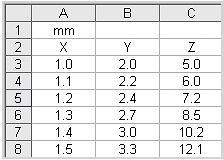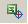# Autodesk Inventor – Import Points from Excel – NACA 4412

Source: Autodesk Inventor

You can import points from a Microsoft® Excel spreadsheet into a 2D sketch, 3D sketch, or drawing sketch. You can use the points to represent items such as inspection points, locations for spot welds, and points on a spline.

The points are automatically created in the sketch according to the coordinates specified in the spreadsheet. The origin of the sketch is used as the origin for the imported point data.

Keep in mind that the points are not associative. A geometry that is based on the points will not be updated if the points are moved or deleted.

The Excel file containing the points should be in the following format:

• The table of points must be in the first worksheet of the Excel file.
• The table always starts at cell A1.
• If the first cell (A1) contains a unit of measure, it is applied to all the points in the spreadsheet. If no unit is specified, the default file units are used.
• Columns are defined as follows:
• Column A is for X coordinates
• Column B is for Y coordinates
• Column C is for Z coordinates
• If the spreadsheet contains Z axis values, only X and Y axis values are imported into a 2D sketch.
• Cells can contain a formula, but the result of the formula must be a numeric value.To import points into a sketch

1. On the Sketch panel bar, click the Import Points icon.2. On the Standard toolbar, click the Point/Center Point tool to specify either sketch points or center points.
3. In the Open dialog box, browse to the folder where the spreadsheet is located, select the spreadsheet, and click Open.

Note: Sketch points are created without constraints. Use the constraint tools to add constraints.